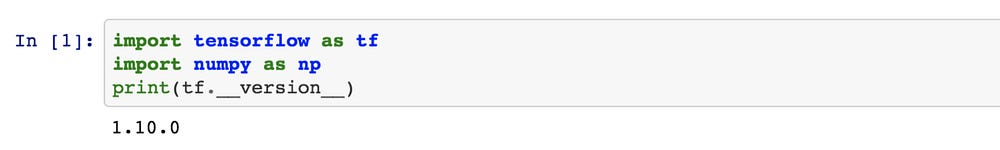Build your first Tensorflow model in 5 minutes!

So you hear about Tensorflow Machine Learning & Deep learning so much and you want to join the party :)

First we should start by setting up the environment.

Let’s first create a directory, run:

`mkdir tf_linear_regression`

Now (recommended) let’s run all in a virtual environment, follow these steps (it works for Mac, if you have Linux or Windows try using Anaconda)

Install Python3

`brew install python3`

(If you don’t have Brew — just install here from here)

Now install the virtual environment

`pip3 install virtualenv`

Actually creating the environment

Go to the directory we’ve created

`cd tf_linear_regression`

`virtualenv -p python3 <path-to-our-dir>`

Now activate it:

`source <path-to-our-dir>/bin/activate`
• To get out of the virtual env run:
`deactivate`

And now for the fun stuff

Install Tensorflow

`pip3 install tensorflow`

Now (just for fun) install Jyputer notebook (you don’t have to, you can copy the code below and paste in a python file and run it

`pip3 install jupyter`

Now let’s try to do something simple & have a look on the equation:

`y = W*x + B`

Assuming we have pairs of inputs and their labels => (X, Y) = [(x1,y1), (x2,y2), (x3,y3),.., (xn,yn)];

i.e for every input x we have the output y. This is supervised machine learning (ML) where for every input we know the desired output.

Now we are going to train W and B in the equation above. Let assume we have (X, Y) = [ (5, 4), (3, 6), (-1, 10)…]

If we are going to guess what W and B would be…. (remember:

y = W*x + B

4 = []*5 +[]; 6 = []*3 + []; 10 = []*(-1) + []. you can see that W = -1 and

B = 9 would be a good fit us…

Now let’s build a model in Tensorflow (TF) which we’ll train to find those values for W and B. the training process in each step would be:

step1: for the input 5 the result is 4 , for the input 3 the result is 6 etc.

You can see that this is the same way we teaching a machine to recognize a cat in a picture: 1. “this input picture is a cat”; 2. “this input picture is a cat”; 3. “this input picture is not a cat” etc.

Let’s START!

Importing TF and Numpy:

Declaring the x, y, w, b:

Declaring the training sets for X and for Y, and declaring the linear equation:

Now we setting the error function (model_delta), the loss function & the optimizer + initializing the variables we declared above:

Now this is a little counter intuitive, but in TF in contrary to program in Python, up until now nothing is running, now we should declare a session and then start the training process:

You can play with the number of iterations, you can see that for 100 we are not even close, but for 1000 it’s much better and 10000 we are nearly there:

• 0.9999 ~ -1
• 8.9998 ~ 9

For the code in Jupyter notebook go to here:
https://github.com/kohn1001/tesorflow-playground/blob/master/linear_regression/linear_regression_tensorflow_basic.ipynb

Source: Deep Learning on Medium